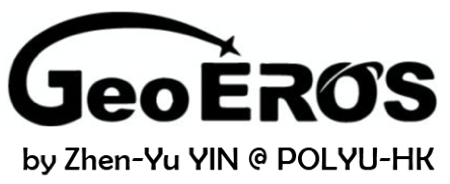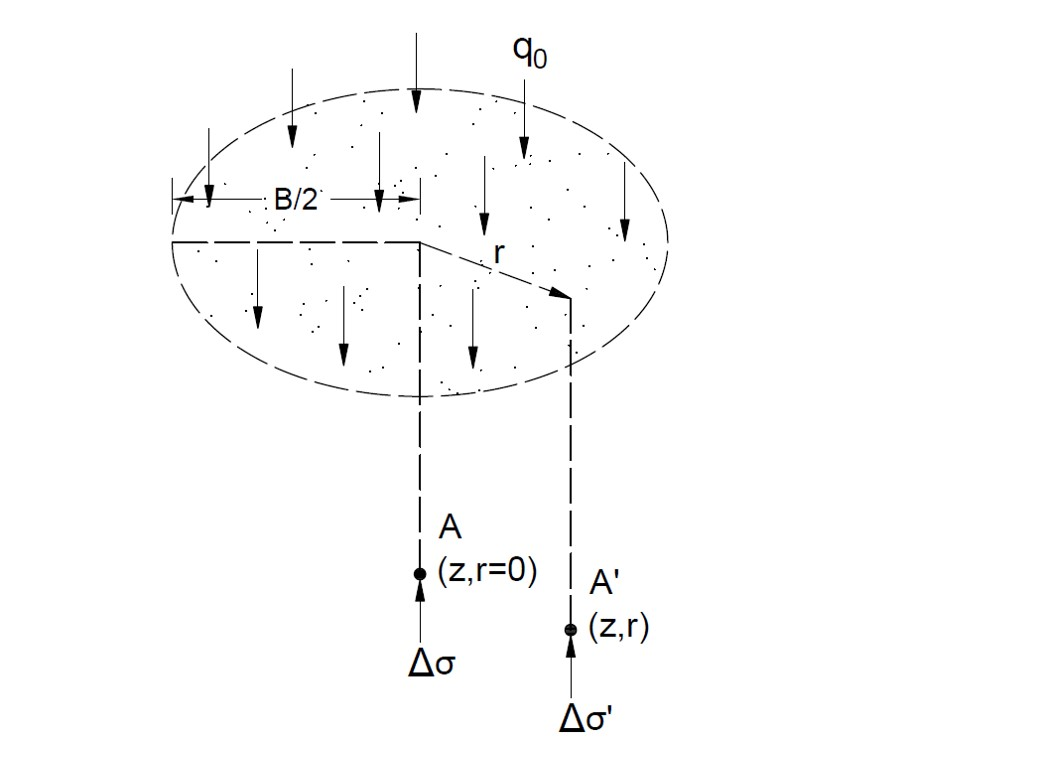# Circular Load Soil Stress Tool## Geometry of Uniform Circular Load## Tips

• This tool is for calculating vertical stress increment in soil induced by the applied uniform circular load, as shown in the figure above.
• The soil layer is assumed as homogeneous, elastic and isotropic.
• All the input parameters should be positive.
• The coordinates of point A are limited in the calculator (z<2B, r<0.5B).
• For "nan", "0" or "inf" displayed in Results, please check your input parameters.

q0 (kN/m2)
B (m)
z (m)
r (m)